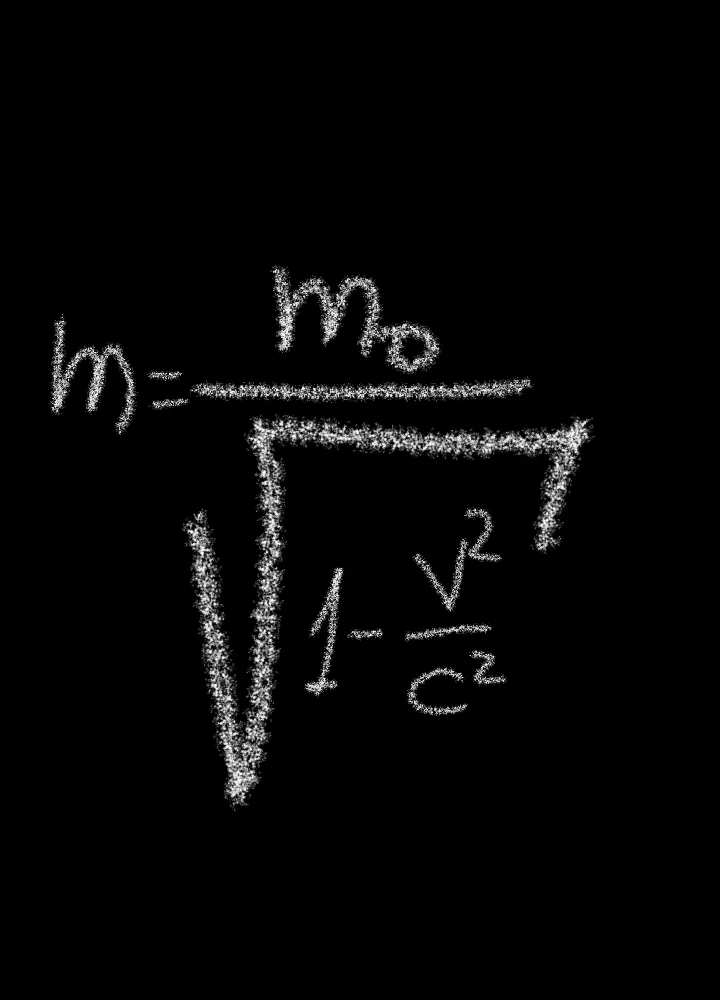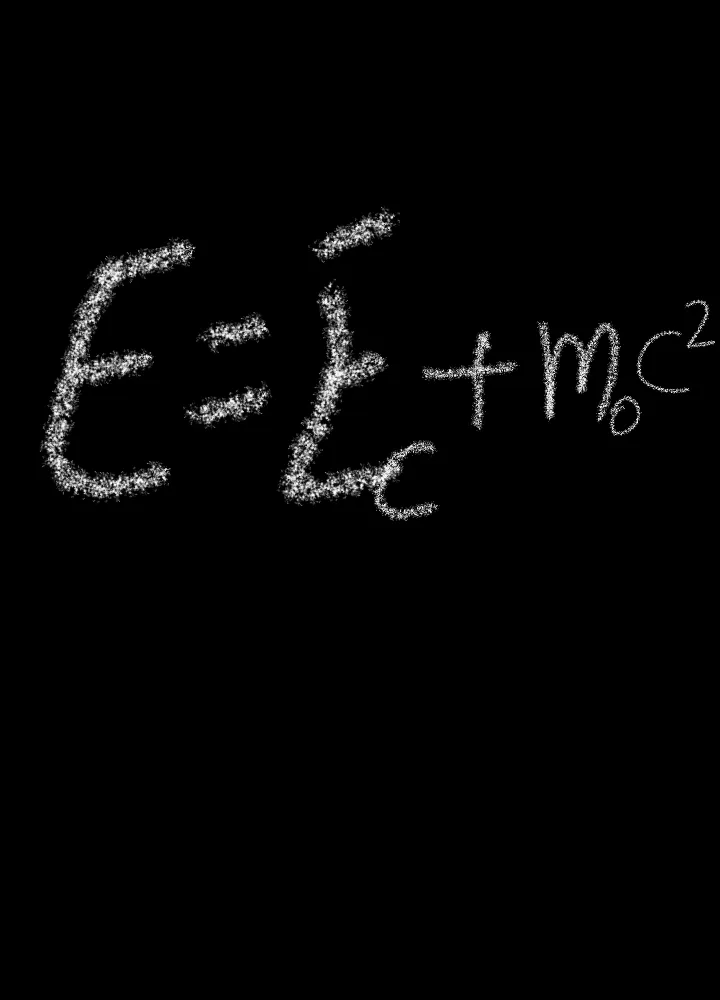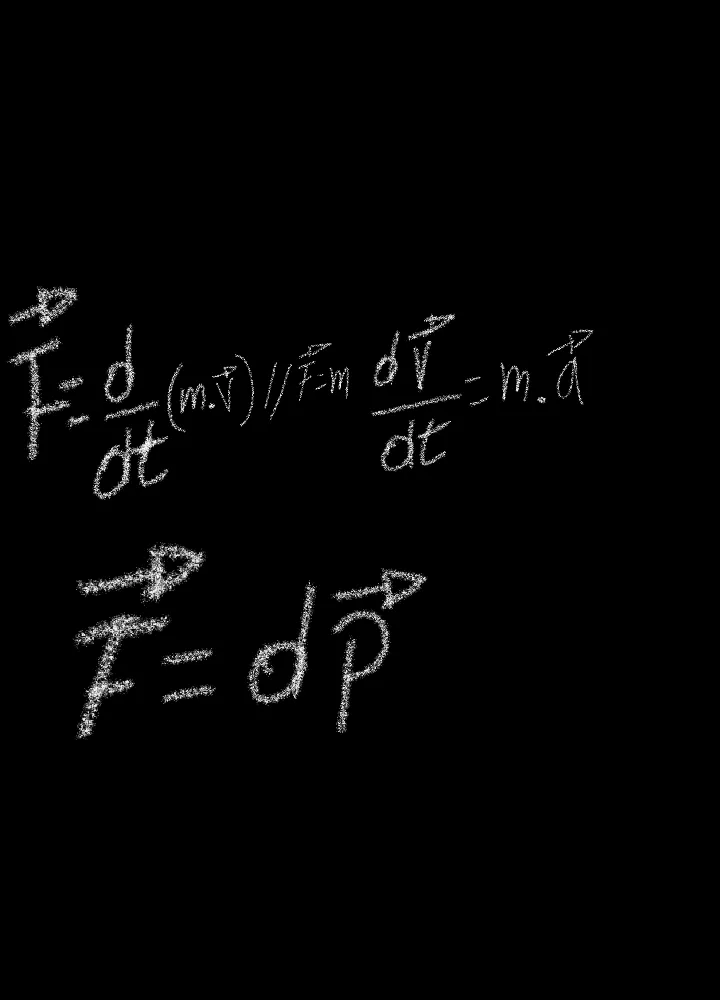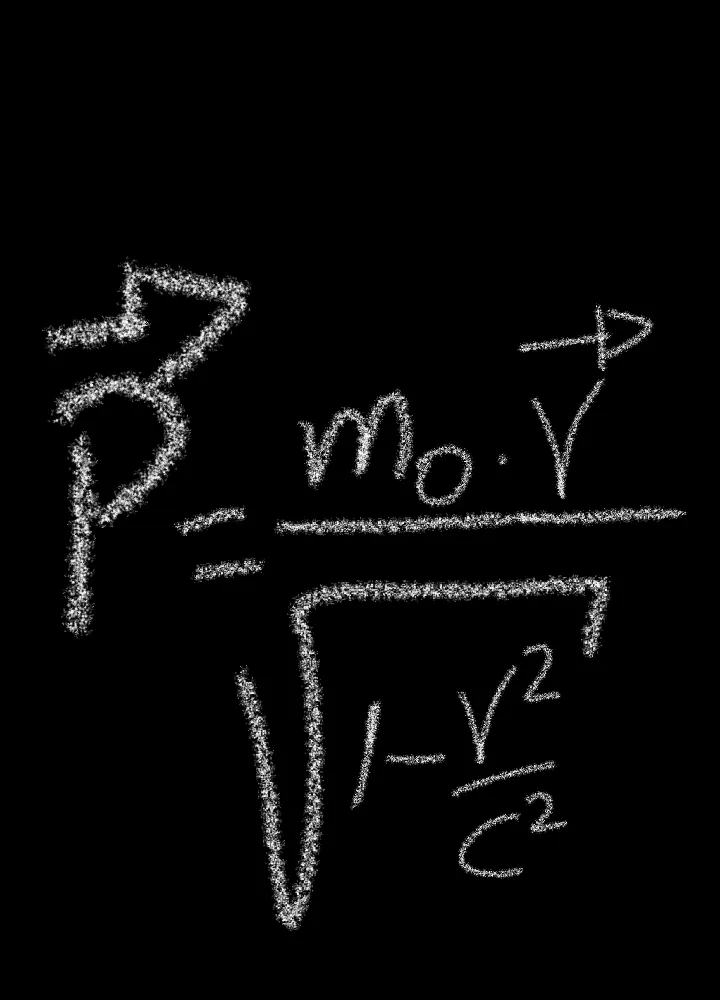# Basics of the principle of conservation

Greetings colleagues from the Hive community, welcome back to my blog, where you can find content to the vast universe of science, mathematics, biology, physics, chemistry, philosophy and general knowledge accompanied by scientific dissemination, a special greetings to communities that it stimulates the content of science

# Introduction

This is another delivery at level of the rifle field, previously shared content at the level of the phenomenon of laluz, in this delivery is made the conceptual relationship over the mass, the catidd of movement, energy, superb reader friend the work, where the relationship of conditions is established, which remains constant until reaching the point of conservation, taking into account that the conservative force, these has to do with those forces whose work, realized is when you move a particle throughout a cycle, considering this closed and null, It is therefore important to analyze, that in the field of physics there are different principles of conservation, but our starting point will be from classical mechanics, because in time they were able to show that the physical changes were not just the distribution of atoms, an example of this is the gravitational force, it is also conservative, which describes its trajectory exercised by a mobile or body.

# Principle of conservation of the mass

In the friend estr dot lover devla science, the most singular of the case is that in carios studies of trial, the atoms to fall in the emptiness these experimented, that this originated a kind of tendency making possible to meet, thanks to the frandes contribution of Lavoiser, by then it was 1789, to be noticed at that time in history, formulates the following law: " The law of conservation of the mass, in which the applied technique argues that the chemical processes, it is affirmed that the sum of reacting mass, this is going to be equal to the mass of the resulting products, but with time I add more content and other posture, because it also applies to the atomic level radiation laws, in physical and chemical process, which it seems, is very singular is complemented with Einstein's theories of relativity, in the dobde the mass of a psrticula this grows with speed. With this mathematical model shows us a (c), is the speed of light with value of 300. 000 km/s, (v) is the particle velocity, (m0) is the resting mass, (m) is the mass which will be measured by an observer, so that it distinguishes its displacement with respect to the speed.# Principle of conservation of energy

In this part of the physicality were managed to by rubbing two bodies these has the ability to produce heat, in order to explain that the certain amount of work, this is able to produce the same amount of heat, as part of molecular kinetica, all these great contributions helped improve the analysis, with the sense that they were able to show that there are several forms of energy such as: elastic, gravitational, thermal, electrical, kinetic and chemistry, relationships were established in the different phenomenos with sense reader friend, that when one type of energy was lost he was manises another cantide and form of energy, ennotro order of ideas were established mathematical models based on the fact of relativistic tepria with respect to the classic, reaching to unify the speed of a particle this is considered despicable in function to the speed of light:As you can see in this simple squaltion, it shows us the mass and energy ratio (m0c-2), which from a mass at rest this can show us the maximum amount of it.

# Principle of conservation of the amount of movement

Having much information on the principle of mass consrvation and energy, with the relationship that it keeps at this point we find, that the force acting on a mass is going to be the same with the same direction as the variation in the amount of movement, starting from the classical equation F-dp/dt, it shows us that (p) is the amount of movement, but that in turn this amount of movement, is defined with p.m.v

But if we establish the following mathematical relationship in which we have the following:In this relationship it is a question of analyzing a system of particles, where the force that acts on each of it can be a type of external force, with meaning that you want to know the amount of movement of a system with the aim of being invariable to 0. Thanks also the law of secservation is associated with the position of Newtonian mechanical, where the system is isolated from all action from the outside, that is why the amount of movement totally should beunaltered concluded in this content shows us if the mass of a particle is growing with speed, apart, also that the theory of relativity shows us several approach, it reaches a point that coincides with the classic when the speed of a particle it's despicable and also thanks to the great work of Lavoisier was able to formulate the law of concervation at the level of chemical processes, giving to explain the sum of the masses that react these will equal the mass of the products.# Consulted Bibliography

[2-Introduction to the circuit studio, by Kendall L. Su, 1979.](https://books.google.co.ve/books?id=3OxAJIzn04EC&pg=PA179&dq=Principios+de+conservaci%C3%B3n&hl=es-419&sa=X&ved=0ahUKEwjC9MCOhsvoAhUlm-AKHV1LA80Q6AEINzAC#v=onepage&q=Principios%20de%20conservaci%C3%B3n&f=false)H2
H3
H4
3 columns
2 columns
1 column